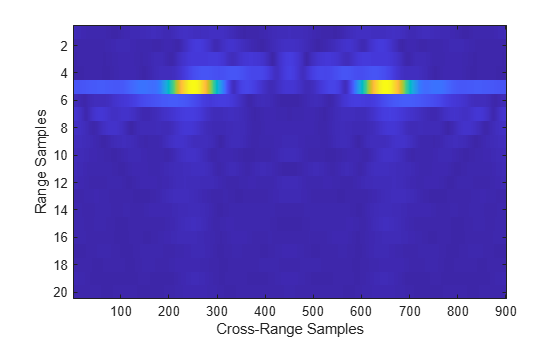# rangeMigrationFMCW

Range migration image formation algorithm for frequency-modulated CW waveform

## Syntax

``slcimg = rangeMigrationFMCW(raw,waveform,fc,v,rc)``
``slcimg = rangeMigrationFMCW(raw,waveform,fc,v,rc,Name=Value)``

## Description

example

````slcimg = rangeMigrationFMCW(raw,waveform,fc,v,rc)` returns a single-look complex image of raw synthetic aperture radar (SAR) data obtained with a frequency modulated continuous wave (FMCW) waveform. The function uses the range migration algorithm.```
````slcimg = rangeMigrationFMCW(raw,waveform,fc,v,rc,Name=Value)` specifies additional inputs using name-value arguments. You can specify the squint angle of the antenna and the signal propagation speed.```

## Examples

collapse all

Load a file that contains simulated unfocused synthetic aperture radar (SAR) data obtained with a frequency modulated continuous wave waveform sampled at 15 MHz. The waveform has a sweep time of 25 microseconds, a sweep bandwidth of 20 MHz, and sweeps in the `"Down"` direction.

```data = load("RangeMigrationFMCWExampleData"); raw = data.raw; wvf = phased.FMCWWaveform(SampleRate=15e6, ... SweepTime=2.5e-5,SweepBandwidth=20e6,SweepDirection="Down");```

The system works at an operating frequency of 3 GHz. The platform moves at a velocity of 200 meters per second and the distance between the beam center on the ground and the radar is 46.672 meters.

```fc = 3e9; v = 200; rc = 46.672;```

Generate a single-look complex image from the data. Plot the image and zoom in on the first 20 along-range samples.

```slcimg = rangeMigrationFMCW(raw,wvf,fc,v,rc); imagesc(abs(slcimg(1:20,:))) xlabel("Cross-Range Samples") ylabel("Range Samples")```## Input Arguments

collapse all

Raw SAR data, specified as a matrix. The data is the unfocused in-phase and quadrature (I/Q) raw data collected by the SAR system. The rows of `raw` correspond to the along-range samples. The columns of `raw` correspond to the pulses received as the platform moves along the cross-range direction. `raw` must have at least two rows.

Data Types: `double`

Input waveform, specified as a `phased.FMCWWaveform` object.

Example: `phased.FMCWWaveform(SweepBandwidth=100e3,OutputFormat="Sweeps",NumSweeps=2)`

Data Types: `double`

Platform velocity, specified as a positive scalar. Units are in meters per second.

Data Types: `double`

Operating frequency, specified as a positive scalar. Units are in Hz.

Example: `2.8e9` Hz specifies a typical S-band operating-frequency value for airport and weather radar systems.

Data Types: `double`

Distance between radar and beam center on the ground, specified as a positive scalar. Units are in meters.

Data Types: `double`

### Name-Value Arguments

Specify optional pairs of arguments as `Name1=Value1,...,NameN=ValueN`, where `Name` is the argument name and `Value` is the corresponding value. Name-value arguments must appear after other arguments, but the order of the pairs does not matter.

Example: `SquintAngle=45,PropagationSpeed=343`

Squint angle of the antenna from the broadside direction in degrees, specified as a scalar in the range `(-90, 90)`.

Data Types: `double`

Signal propagation speed in meters per second, specified as a positive scalar.

Example: `343` meters per second approximates the speed of sound at sea level and at a temperature of 20 °C under normal atmospheric conditions.

Data Types: `double`

## Output Arguments

collapse all

Single-look complex (SLC) image, returned as a matrix. `slcimg` is the same size as `raw` and contains the focused data processed by the range migration algorithm.

## Version History

Introduced in R2022a